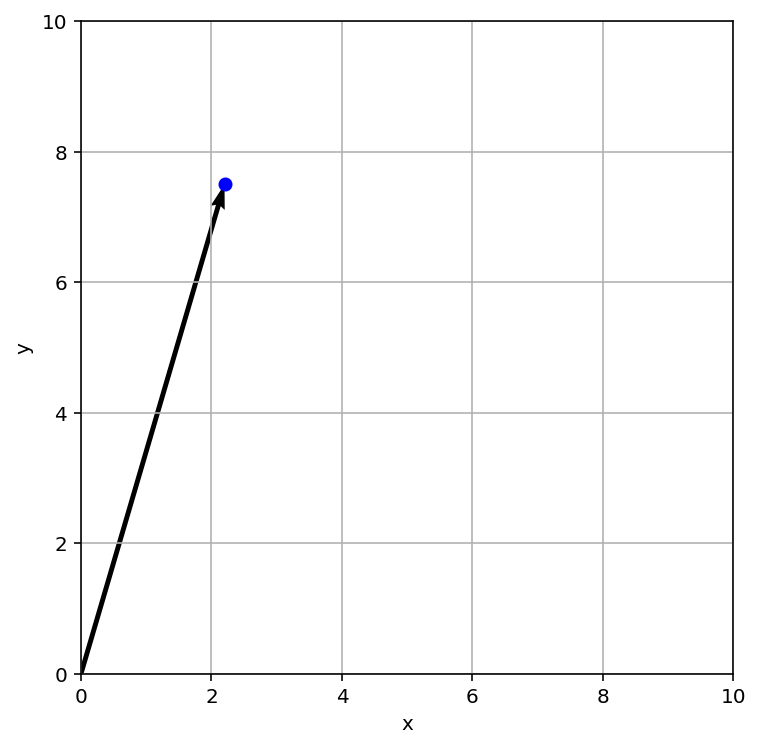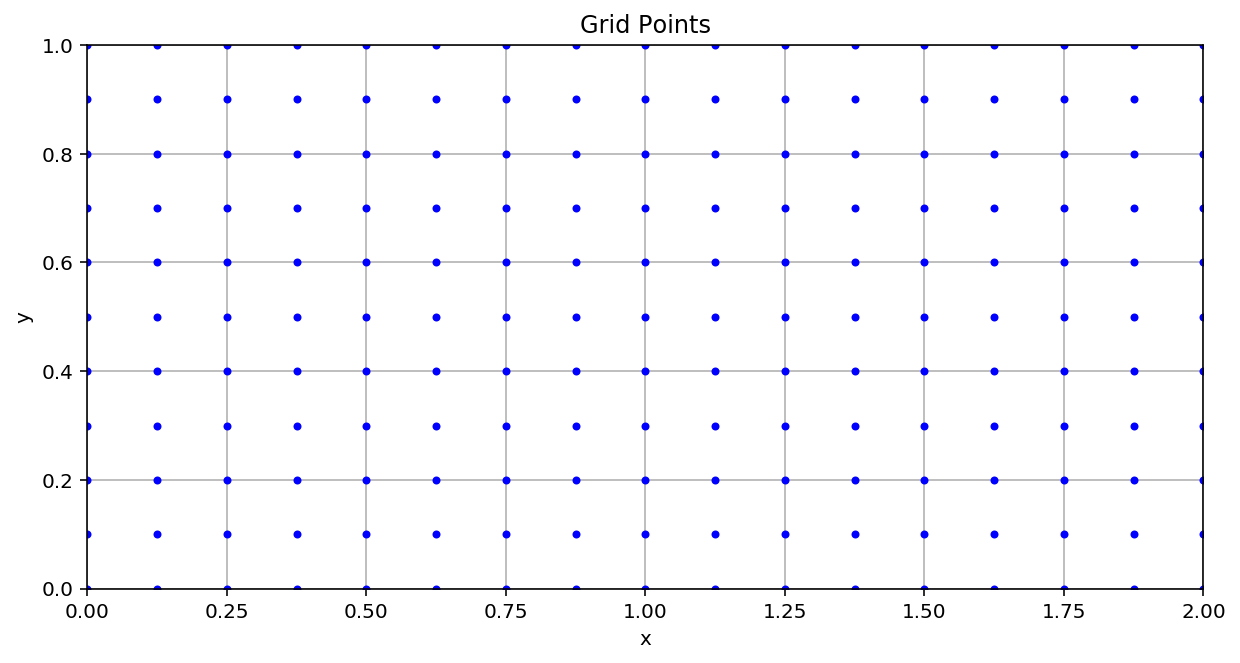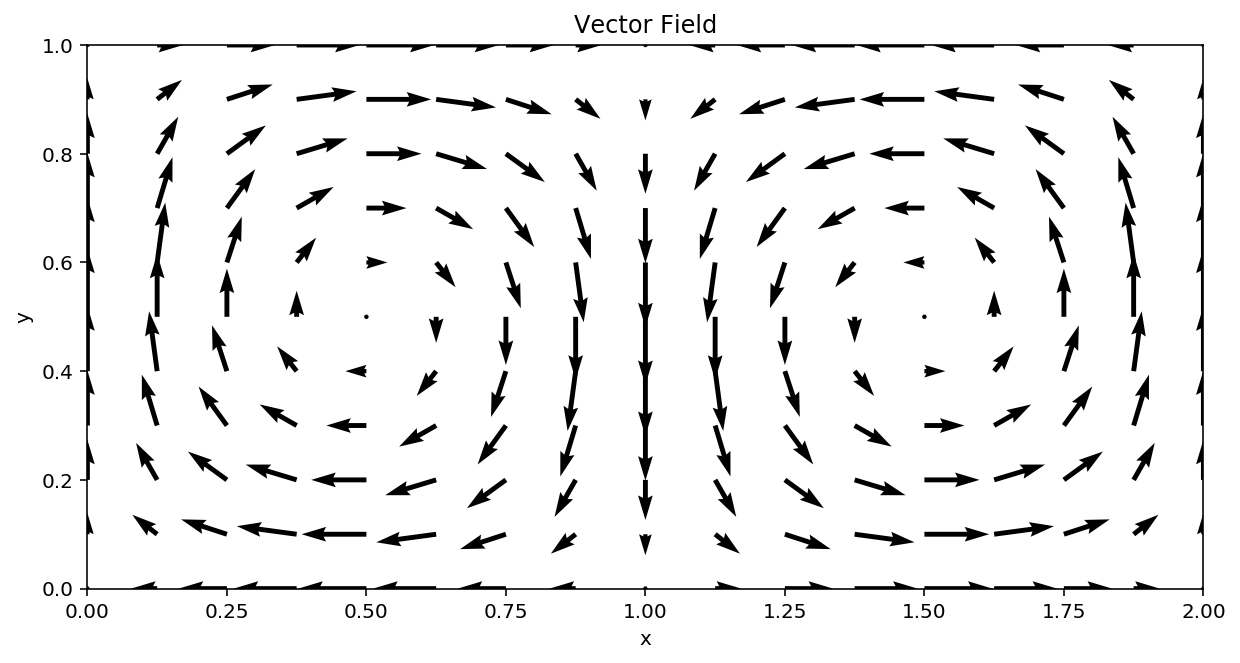# Fluid Kinematics¶

## Learning Outcomes¶

This Jupyter Notebook will introduce ideas related to how fluid motion can be described mathematically. These are very important tools that let engineers build systems and machines that involve fluid flow. Examples are vehicles of all kinds, pumps and engines, weather simulations, domestic plumbing systems and medical implants.

The following concepts will be discussed in detail:

• Velocity fields
• Eulerian and Lagrangian descriptions of fluid motion.
• Streamlines, pathlines, and streaklines

Most of you should be familiar with the idea of a vector field already but it's always a good idea to take a fresh look. Concepts such as Eulerian and Lagrangian descriptions might seem abstract at first but they are important ideas in taming the complex nature of fluid flow which allows us to work as engineers. In the next notebook we will look at the Reynolds Transport Theorem, which will allow us to move from the Lagrangian to the Eulerian description. As you will see this will make analysis of many flows much easier.

You will no doubt have heard the term 'streamlined' before and perhaps have a feeling for what it means. It might be along the lines that a race car is streamlined while a bus or a lorry is not. Here we will describe what streamlines mean mathematically and also introduce the related ideas of pathlines and streaklines and how we can use them to describe fluid flow.

## Vector fields¶

Let take a step back from this complexity and deal with some of the basics.

Take a look at the weather map of Ireland below. Each icon on this map is fixed in space (longitude and latitude) and the pointy end indicates the direction of the wind. The numeric value indicates the speed of the wind. Therefore the icon gives us the local velocity vector. A field of these vectors arranged over a space is a vector field.A simple vector field in the form of a weather forecast, source [met.ie](https://www.met.ie)

To implement this mathematically we first need to define the positions of the vectors in our space. We can define a field of coordinates in 3D ($\mathbb{R}^3$) space as $\vec{x}(x,y,z)$; a series of vectors each of which point from the origin to a point $(x,y,z)$. Note these are vectors that simply point to positions in space and are not velocity vectors.

We can write these position vectors using the idea of unit vector notation where $(\hat{i},\hat{j},\hat{k} )$ are, as the name implies, unit length vectors along the axes of the coordinate system. They can then be multiplied by scalers to transform them to any point in $\mathbb{R}^3$. We can write this as follows:

\begin{align} \vec{{x}} (x,y,z) = x \hat{i} + y \hat{j} + z \hat{k} \end{align}

So as we change the values of $x$, $y$ or $z$ we can control where the transformed unit vector points.

Lets look at an interactive example in 2D or $\mathbb{R}^2$.

In :
import numpy as np
import matplotlib.pyplot as plt

%matplotlib inline
%config InlineBackend.figure_format = 'retina'

# The first thing we need to do is select the values of our scalers x and y that determine where our point in space is.
x = 2.2 # Edit this number to any value between 0 and 10 for x
y = 7.5 # Edit this number to any value between 0 and 10 for x

# Now lets make a plot to draw on
fig, ax = plt.subplots(figsize=(6,6))

# Plot the (x,y) location as a blue circle
ax.plot(x,y,'bo')
# Draw an arrow from the origin to the point (x,y) using the quiver command: ax.quiver(x, y)
# Note the extra code to control the lenght of the arrow.
ax.quiver(x, y, scale_units='xy', scale=1)

# This just sets the upper and lower limits on the plot from 0 to 10.
plt.xlim([0, 10])
plt.ylim([0, 10])

# Add text labels to the axes
plt.xlabel("x")
plt.ylabel("y")

# Draw gridlines
plt.grid()

# Fix the plot aspect ratio
ax.set_aspect('equal')

# Finally display the plot below
plt.show()We now have a way to select any point in space using unit vector notation. This gives us location to put our wind vectors as in the map above. What about the velocity at each point? Do to this we need to define a vector $\vec{V}$ that is a function of every point $\vec{x}$ but also for time $t$ since the wind can change over the course of the day as well as from location to location. This looks like:

\begin{align*} \vec{V}(\vec{x},t) = u \hat{i} + v \hat{j} + w \hat{k} \end{align*}

Note that now we have new scalers $(u,v,w)$ These are the velocity components along each axis of the coordinate system. So at some point $\vec{x}$ and time $t$ we have a velocity $\vec{V}$ that has a component $u$ in the $i$ direction, $v$ in the $j$ direction and $w$ in the $k$ direction.

Lets look at another $\mathbb{R}^2$ example with Python. This time we will define a grid of points.

In :
# We've already imported the required libraries, nunpy and matplotlib in the prevous cell.

# Lets start by creating a grid of points (X,Y)

# the np.arrange function simply creates a list of numbers.
# for example:
# np.arange(0,10,2)
# returns:
# array([0, 2, 4, 6, 8])
# we can define a range of points along the x and y axis as follows:
x = np.arange(0, 2.1, 0.125) # from 0 up to 2.1 in steps of 0.125. (note that 2.1 is not included)
y = np.arange(0, 1.1, 0.1)

# However we want a 2D grid of numbers so we can use the command meshgrid to fill it in.
X, Y = np.meshgrid(x, y)

# Create a new figure axes
fig, ax1 = plt.subplots(figsize=(10,5))

# Plot the grid points (X,Y)
ax1.plot(X,Y,'b.')

plt.xlabel("x")
plt.ylabel("y")
plt.title("Grid Points")
ax.set_aspect('equal')
plt.grid()
plt.xlim([0, 2])
plt.ylim([0, 1])
plt.show()

# Now lets define values of local velocity with components u and v for each point (X,Y).
# By using trigonometric relations relative to the values of (X,Y) we can get a nice result.
u = -np.sin(np.pi * X) * np.cos(np.pi * Y)
v =  np.cos(np.pi * X) * np.sin(np.pi * Y)

# Create a new figure
fig, ax2 = plt.subplots(figsize=(10,5))

# Plot the result using the quiver function which is designed to plot vector fields.
ax2.quiver(X, Y, u, v)

plt.xlabel("x")
plt.ylabel("y")
plt.title("Vector Field")
plt.xlim([0, 2])
plt.ylim([0, 1])
ax.set_aspect('equal')You may have noticed that nowhere in the above example did we use $\hat{i}$ or $\hat{j}$. In fact the tools provided by the matpotlib library handle this for us and we just need to tell it the scalers $(x,y)$ or $(u,v)$.

Here the velocity field is given by the simple expressions:

\begin{align*} u &= &-\sin(\pi x) \cos(\pi y)\\ v &= &\cos(\pi x) \sin(\pi y) \end{align*}

This results in a double-vortex flow field and the flow is observed to swirl around. This is similar to the flow behind an aircraft which we will learn a lot more about in Mechanics of Fluids II.*Wing tip vortices trailing a light aircraft, source [NASA Langley Research Center](https://howthingsfly.si.edu/media/wing-tip-vortex)*

So what does all this mean? What do all these arrows tell us?

If you consider any point in the flow, the vector's length and orientation at that point tells you the speed and direction of the flow at time $t$. Just like the symbols in the weather forecast. You can think of the vector field above as a single frame from a video. If the flow changes in time (what is referred to as unsteady flow) the length and orientation of the vectors will change as the video plays. Note however that the points $(x,y)$ where the tails of the vectors begin will not change.

Lets looks at a simple example of the airplane. The flow over the airplane is 3D and complex but we can look at 2D slices of the flow to get a understanding of what is happening. You can imagine that this 2D slice is following along behind the airplane as it flies with some velocity $\vec{V}$ or the airplane is a model in a wind tunnel. If we only look at the velocity parallel to the plane ($\vec{V}(y,z)$) then we might see a flow pattern as shown. The flow velocity normal to the 2D slice is not considered right now.*A vector field in a 2D slice located behind an airplane*

Why do this? There are a number of reasons why we would want to look at fluid flow in this way. One obvious reason is measurement. If we want to measure the flow velocity behind a model in a wind tunnel we could position a probe at various locations in a grid. Similarly we can arrange weather monitoring stations around the country to measure the wind velocity field. We can also measure the temperature and pressure scalar fields too.

This description of the flow as a vector field at fixed points in space is the Eulerian description of flow. Points are fixed in space and the change in some quantity, such as velocity, is tracked at these points as the fluid passes over each point.

It was mentioned above that the velocity field is a function of space and time $\vec{V}(\vec{{}x},t)$. SO far we have only considered space and assumed that the flow is steady, or invariant in time. This is often the case in many types of flows, however most flows we observe are not time invariant. The blood flow pulsing in the body is a good example. Lets look again at our Python code for plotting vector maps and introduce some time dependence. This cell will take a few seconds to run because it first has to be recorded into a HTML format.

In :
# To show the effect of time we need to animate the vector map so import the matplotlib animation library.
from matplotlib import animation
# We can use HTML5 to make a nice embedded video, IPython is just an old name for the Jupyter project.
from IPython.display import HTML

fig, ax = plt.subplots(figsize=(10,5))

# Lets reuse a lot of code
x = np.arange(0, 2.1, 0.125)
y = np.arange(0, 1.1, 0.1)

X, Y = np.meshgrid(x, y)

u = -np.cos(np.pi * X) * np.cos(np.pi * Y)
v =  np.cos(np.pi * X) * np.sin(np.pi * Y)

ax.plot(X,Y,'bo')
# Note that now we output quiver to an object Q that lets us edit the properties and contents of the plot.
Q = ax.quiver(X, Y, u, v)

plt.xlim([0, 2])
plt.ylim([0, 1])
plt.grid()

# We dont want to plot the vector field but offload it to a HTML video
plt.close()

# def allows us to define a funciton. This is a piece of code we which to reuse again and again.
# in this case we want to contineously update the values of (u,v) as they are modified in time.
# Pay attention to the syntax used. The indenting determines what lines of code are included in the function
def update_quiver(t, Q, X, Y):
# To make the flow change with time we add a term: 0.5*np.sin(t*0.25)) to perturb the vector field
u = -np.sin(np.pi * X + 0.5*np.sin(t*0.25)) * np.cos(np.pi * Y)
v =  np.cos(np.pi * X + 0.5*np.sin(t*0.25)) * np.sin(np.pi * Y)
Q.set_UVC(u, v)
return (Q,)

# This code is used to generate the animation
anim = animation.FuncAnimation(fig, update_quiver, fargs=(Q, X, Y), interval=100, blit=True)

# This outputs it to a nifty video player
HTML(anim.to_html5_video())

Out: